# 1685 Oh, No! I’ve Created a Monster!

### Today’s puzzle:

You may see some Frankenstein monsters walking about this time of year, but there’s no reason to be afraid of them or of this monster puzzle I’ve created. Simply write the numbers 1 to 12 in both factor areas so that the puzzle functions like a multiplication table.Here’s the same puzzle without any added color, if that’s what you prefer:### Factors of 1685:

• 1685 is a composite number.
• Prime factorization: 1685 = 5 × 337.
• 1685 has no exponents greater than 1 in its prime factorization, so √1685 cannot be simplified.
• The exponents in the prime factorization are 1 and 1. Adding one to each exponent and multiplying we get (1 + 1)(1 + 1) = 2 × 2 = 4. Therefore 1685 has exactly 4 factors.
• The factors of 1685 are outlined with their factor pair partners in the graphic below.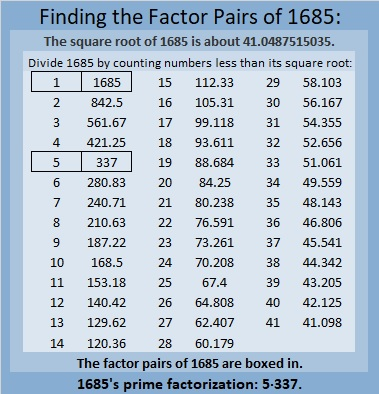### More About the Number 1685:

1685 is the sum of two squares in two different ways:
41² + 2² = 1685, and
34² + 23² = 1685.

1685 is the hypotenuse of FOUR Pythagorean triples:
164-1677-1685, calculated from 2(41)(2), 41² – 2², 41² + 2²,
627-1564-1685, calculated from 34² – 23², 2(34)(23), 34² + 23²,
875-1440-1685, which is 5 times (175-288-337), and
1011-1348-1685, which is (3-4-5) times 337.

# 1683 Grave Marker

### Today’s Puzzle:

It’s almost Halloween. I hope you enjoy this grave-marker puzzle. Write the numbers from 1 to 12 in both the first column and the top row so that those numbers and the given clues make a multiplication table.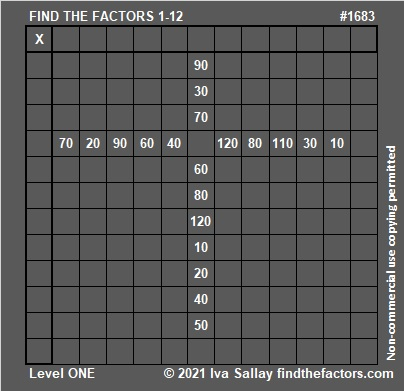Here’s the same puzzle, but it won’t use up all your printer ink.### Factors of 1683:

• 1683 is a composite number.
• Prime factorization: 1683 = 3 × 3 × 11 × 17, which can be written 1683 = 3² × 11 × 17.
• 1683 has at least one exponent greater than 1 in its prime factorization so √1683 can be simplified. Taking the factor pair from the factor pair table below with the largest square number factor, we get √1683 = (√9)(√187) = 3√187.
• The exponents in the prime factorization are 2, 1, and 1. Adding one to each exponent and multiplying we get (2 + 1)(1 + 1)(1 + 1) = 3 × 2 × 2 = 12. Therefore 1683 has exactly 12 factors.
• The factors of 1683 are outlined with their factor pair partners in the graphic below.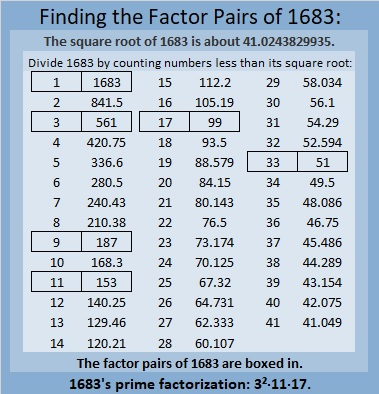### More About the Number 1683:

1683 is the hypotenuse of a Pythagorean triple:
792-1485-1683, which is (8-15-17) times 99.

1683 is the difference of two squares in SIX different ways:
842² – 841² = 1683,
282² – 279² = 1683,
98² – 89² = 1683,
82² – 71² = 1683,
58² – 41² = 1683, and
42² – 9² = 1683.
That last one means we are 81 numbers away from the next perfect square. I also highlighted a cool-looking difference.

1680, 1681, 1682, 1683, and 1684 are the second smallest set of FIVE consecutive numbers whose square roots can be simplified.# 1678 and Level 5

### Today’s Puzzle:

Using logic, write the numbers from 1 to 10 in both the first column and the top row so that this puzzle will function like a multiplication table.### Factors of 1678:

• 1678 is a composite number.
• Prime factorization: 1678 = 2 × 839.
• 1678 has no exponents greater than 1 in its prime factorization, so √1678 cannot be simplified.
• The exponents in the prime factorization are 1 and 1. Adding one to each exponent and multiplying we get (1 + 1)(1 + 1) = 2 × 2 = 4. Therefore 1678 has exactly 4 factors.
• The factors of 1678 are outlined with their factor pair partners in the graphic below.### More about the number 1678:

Because 2(839)(1) = 1678, you can calculate the only Pythagorean triple that contains the number 1678:
The smaller leg will be 2(839)(1) = 1678.
The longer leg will be 839² – 1² = 703920.
The hypotenuse will be 839² + 1² = 703922.

# 1675 You CAN Solve This Level 3 Puzzle!

Great news for math enthusiasts, students, and teachers everywhere! The #148 Playful Math Education Carnival was published today at Math Book Magic!

### Today’s puzzle:

I am confident that you can solve this level 3 puzzle! Here’s how: Using only the numbers from 1 to 10, write the factors of 27 and 6 in the appropriate cells. Next, write 18’s factors. Then, since this is a level 3 puzzle, write the factors of 30, 50, 40, 36, 14, 56, and 8, in that order, until all the numbers from 1 to 10 appear in the first column as well as in the top row. As always, there is only one solution.What did I tell you? You could solve it!

### Factors of 1675:

• 1675 is a composite number.
• Prime factorization: 1675 = 5 × 5 × 67, which can be written 1675 = 5² × 67.
• 1675 has at least one exponent greater than 1 in its prime factorization so √1675 can be simplified. Taking the factor pair from the factor pair table below with the largest square number factor, we get √1675 = (√25)(√67) = 5√67.
• The exponents in the prime factorization are 2 and 1. Adding one to each exponent and multiplying we get (2 + 1)(1 + 1) = 3 × 2 = 6. Therefore 1675 has exactly 6 factors.
• The factors of 1675 are outlined with their factor pair partners in the graphic below.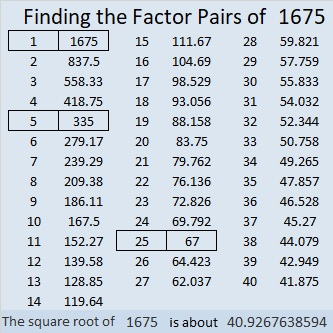### More About the Number 1675:

Did you notice that 1675 = 5 × 67 × 5?

How many quarters are in \$16.75?
Well, 17 × 4 quarters = 68 quarters = \$17.00.
Subtracting one quarter from both sides of the equation, we get
67 quarters = \$16.75.

1675 is the hypotenuse of TWO Pythagorean triples:
469-1608-1675, which is (7-24-25) times 67, and
1005-1340-1675, which is (3-4-5) times 335.

1675 is the difference of two squares in THREE different ways:
838² – 837² =  1675,
170² – 165² =  1675, and
46² – 21²  =  1675.

# 1674 and Level 2

### Today’s Puzzle:

Make a multiplication table out of this puzzle. Can you see how to do it? The factors won’t be in the usual order, but I’m sure you can figure it out!### Factors of 1674:

• 1674 is a composite number.
• Prime factorization: 1674 = 2 × 3 × 3 × 3 × 31, which can be written 1674 = 2 × 3³ × 31.
• 1674 has at least one exponent greater than 1 in its prime factorization so √1674 can be simplified. Taking the factor pair from the factor pair table below with the largest square number factor, we get √1674 = (√9)(√186) = 3√186.
• The exponents in the prime factorization are 1,3 and 1. Adding one to each exponent and multiplying we get (1 + 1)(3 + 1)(1 + 1) = 2 × 4 × 2 = 16. Therefore 1674 has exactly 16 factors.
• The factors of 1674 are outlined with their factor pair partners in the graphic below.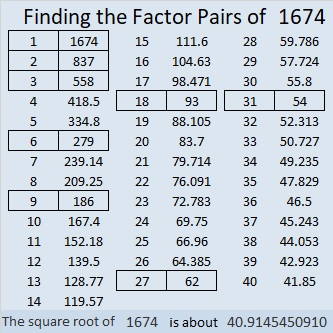### More about the number 1674:

From OEIS.org we learn that
1/1 + 1/2 + 1/3 + 1/4 + . . . + 1/1672 + 1/1673 ≈ 7.999888, but if you add the next tiny fraction, 1/1674, the sum will be a tiny bit more than 8 or approximately 8.000486.

That’s adding a whole lot of unit fractions just to get a sum over 8.

# 1673 and Level 1

### Today’s Puzzle:

Write the numbers from 1 to 10 in both the first column and the top row so that those numbers and the given clues become a not-in-the-usual-order multiplication table.### Factors of 1673:

• 1673 is a composite number.
• Prime factorization: 1673 = 7 × 239.
• 1673 has no exponents greater than 1 in its prime factorization, so √1673 cannot be simplified.
• The exponents in the prime factorization are 1 and 1. Adding one to each exponent and multiplying we get (1 + 1)(1 + 1) = 2 × 2 = 4. Therefore 1673 has exactly 4 factors.
• The factors of 1673 are outlined with their factor pair partners in the graphic below.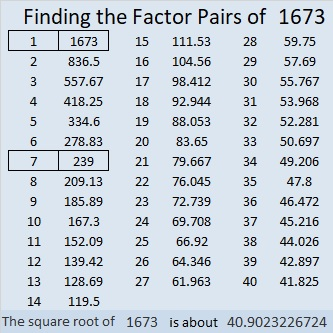### More About the Number 1673:

The factors of 1673 are 1, 7, 239, and 1673. OEIS.org tells us something cool about the sum of the squares of those factors:
1² + 7² + 239² + 1673² = 1690².

# 1672 A Pythagorean Triple Puzzle with Only Two Clues

### Today’s Puzzle:

If you would like to try a Pythagorean Triple Puzzle that isn’t too difficult, I’ve got you covered with today’s puzzle:

PUZZLE DIRECTIONS: This puzzle is NOT drawn to scale. Although all of the angles may look like 60-degree angles, none of them are. The marked angles are 90 degrees. Lines that look parallel are NOT parallel. Although side lengths look equal, they are NOT equal. Most rules of geometry do not apply here. When drawn to scale, none of the triangles in this particular puzzle overlap, but that is not always the case for some of my other Pythagorean triple puzzles.

No geometry is needed to solve this puzzle. All that is needed is logic and the table of Pythagorean triples under the puzzle. The puzzle only uses triples in which each leg and each hypotenuse is less than 100 units long. The puzzle has only one solution.You can also print and cut out the triangle cards below to solve the puzzle.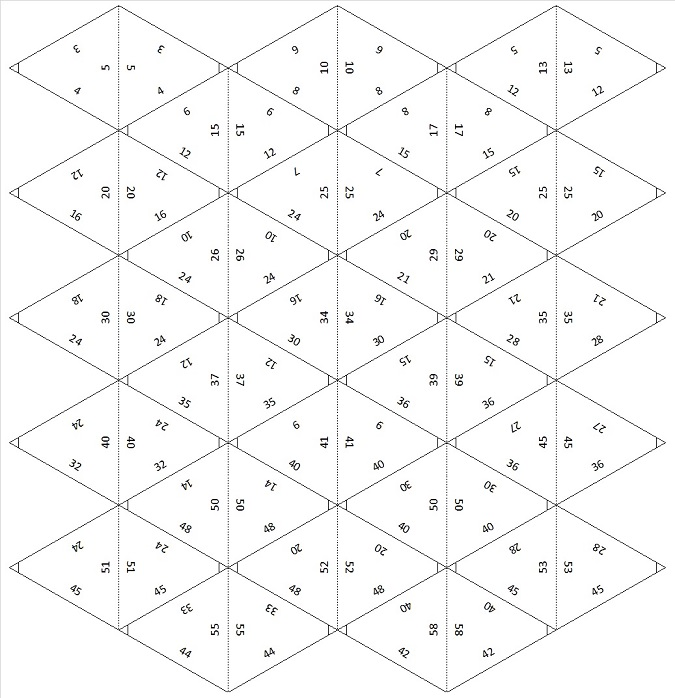### Factors of 1672:

• Prime factorization: 1672 = 2 × 2 × 2 × 11 × 19, which can be written 1672 = 2³ × 11 × 19.
• 1672 has at least one exponent greater than 1 in its prime factorization so √1672 can be simplified. Taking the factor pair from the factor pair table below with the largest square number factor, we get √1672 = (√4)(√418) = 2√418.
• The exponents in the prime factorization are 3,1 and 1. Adding one to each exponent and multiplying we get (3 + 1)(1 + 1) (1 + 1) = 4 × 2 × 2 = 16. Therefore 1672 has exactly 16 factors.
• The factors of 1672 are outlined with their factor pair partners in the graphic below.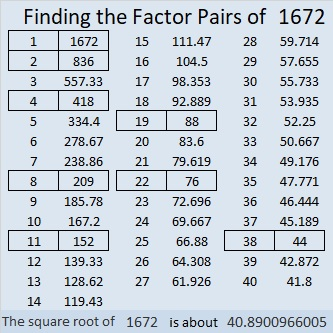### More About the Number 1672:

1672 is the difference of two squares in FOUR different ways:
419² – 417² = 1672,
211² – 207² = 1672,
49² – 27² = 1672, and
41² – 3² = 1672.
That last one means we are only 9 numbers away from the next perfect square, 1681.

From NumbersaPlenty, we learn that 1672 is a digitally powerful number:
1672 = 1¹ + 6⁴ + 7³ + 2⁵.

# 1671 Are Vaccines Really Much of a Mystery?

### Today’s Puzzle:

Why is this puzzle a mystery-level puzzle? As long as you don’t make any assumptions, it really isn’t all that difficult. Study all the clues. Some of them are tricky, but there is ONE logical place to start that won’t make you guess, check, erase and try again. Don’t let any other clues fool you into thinking you should start with them. Find that one logical place to start, then write the numbers from 1 to 14 in both the first column and the top row so that those numbers and the given clues create a multiplication table. As always, there is only one solution.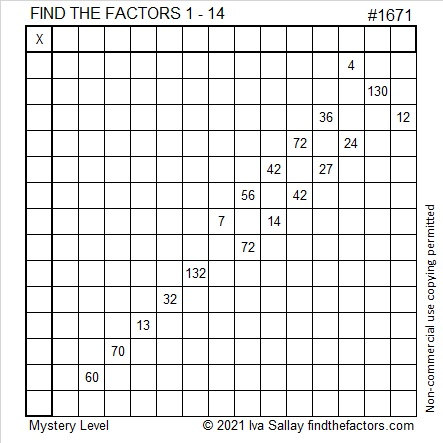Getting vaccinated against COVID is also not much of a mystery as long as you don’t make any dangerous assumptions. I am grateful that I received my doses of the Pfizer vaccine on 22 February and 15 March 2021.

My daughter’s brother-in-law was a healthy police officer who started having difficulty breathing. When it got worse, he was hospitalized. Before long he had to be put into a coma. He had significant lung fibrosis due to COVID complications. Even if he could recover, his life would never again be what it used to be. A few days after he was hospitalized, his wife and children also came down with COVID, so they were no longer allowed to visit him in the hospital.  His coworkers asked his wife what they could do for her. Without reservation, she answered, “Get vaccinated!” The doctor video-chatted with his family and told them to start thinking about preparing themselves for his death. On a Sunday afternoon, they made a video call as his mother held up the phone for him in the hospital. They said goodbye, but how much does a person in a coma hear? How satisfying is a video call for a final goodbye?

On Tuesday, there seemed to be hope. A new doctor felt that while he wasn’t getting any better, he wasn’t getting any worse either. His heart was still strong and his other organs were working. There was still hope. If he got better, he could get a lung transplant. A miracle could still happen.

By Thursday, other organs began to fail, and all hope was gone. His family had recovered enough to visit him in the hospital to say their final goodbyes. It was better than a video call but still terribly heartbreaking.

Although I had probably only met him once, seven years ago when my daughter married into his wife’s family, I spent a lot of time crying when I got the news.

His funeral will be tomorrow. He was the first active-duty police officer in my state to die from COVID 19. Here his wife describes what a great loss his death is to his community and to their family. She pleads with everyone to take the virus seriously and get vaccinated.

Please, do everything you can to protect yourself and others from COVID 19. Get vaccinated. Social distance as best you can. Wear a mask.

### Factors of 1671:

This is my 1671st post, so I’ll share the factors of 1671:

• 1671 is a composite number.
• Prime factorization: 1671 = 3 × 557.
• 1671 has no exponents greater than 1 in its prime factorization, so √1671 cannot be simplified.
• The exponents in the prime factorization are 1 and 1. Adding one to each exponent and multiplying we get (1 + 1)(1 + 1) = 2 × 2 = 4. Therefore 1671 has exactly 4 factors.
• The factors of 1671 are outlined with their factor pair partners in the graphic below.### More About the Number 1671:

1671 is the hypotenuse of a Pythagorean triple:
495-1596-1671 which is 3 times (165-532-557).

# 1670 Mystery Puzzle

### Today’s Puzzle:

The logic needed to solve this puzzle is a bit complicated. If you would like a hint, I’ll give one later in the post that will remove most of the mystery in the puzzle. Write the numbers 1 to 12 in both the first column and the top row so that those numbers and the given clues become a multiplication table.Logic Hint:
The 33 means that the 9 cannot be 3 × 3, so the 9 and the 54 must use both 9’s.
The 54 also must use a 6.
Thus the 36 near the bottom of the puzzle cannot be 9 × 4 or 6 × 6 and must be 3 × 12.
84 and that bottom 36 must use both 12’s, so the 48 must be 6 × 8 and not 12 × 4.
The rest of the puzzle should be rather straightforward.

### Factors of 1670:

• 1670 is a composite number.
• Prime factorization: 1670 = 2 × 5 × 167.
• 1670 has no exponents greater than 1 in its prime factorization, so √1670 cannot be simplified.
• The exponents in the prime factorization are 1, 1, and 1. Adding one to each exponent and multiplying we get (1 + 1)(1 + 1)(1 + 1) = 2 × 2 × 2 = 8. Therefore 1670 has exactly 8 factors.
• The factors of 1670 are outlined with their factor pair partners in the graphic below.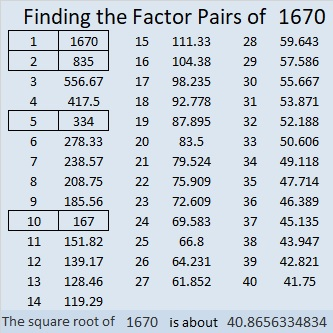### More About the Number 1670:

1670 is the hypotenuse of a Pythagorean triple:
1002-1336-1670, which is (3-4-5) times 334.

Although it is very much irrational, OEIS.org informs us that the first few digits of
1670^(1/6) is 3.44444624848…

# 1669 and Level 6

### Today’s Puzzle:

Some sets of clues in this puzzle have two possible common factors, and another set has three possible common factors. Don’t guess which ones to use, but use logic instead! Write the numbers 1 to 12 in both the first column and the top row so that those numbers and the given clues form a multiplication table.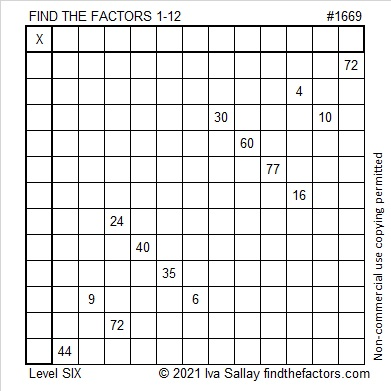### Factors of 1669:

• 1669 is a prime number.
• Prime factorization: 1669 is prime.
• 1669 has no exponents greater than 1 in its prime factorization, so √1669 cannot be simplified.
• The exponent in the prime factorization is 1. Adding one to that exponent we get (1 + 1) = 2. Therefore 1669 has exactly 2 factors.
• The factors of 1669 are outlined with their factor pair partners in the graphic below.How do we know that 1669 is a prime number? If 1669 were not a prime number, then it would be divisible by at least one prime number less than or equal to √1669. Since 1669 cannot be divided evenly by 2, 3, 5, 7, 11, 13, 17, 19, 23, 29, 31, or 37, we know that 1669 is a prime number.

### More About the Number 1669:

1669 is the sum of two squares:
38² + 15² = 1669.

1669 is the hypotenuse of a primitive Pythagorean triple:
1140-1219-1669, calculated from 2(38)(15), 38² – 15², 38² + 15².

Here’s another way we know that 1669 is a prime number: Since its last two digits divided by 4 leave a remainder of 1, and 38² + 15² = 1669 with 38 and 15 having no common prime factors, 1669 will be prime unless it is divisible by a prime number Pythagorean triple hypotenuse less than or equal to √1669. Since 1669 is not divisible by 5, 13, 17, 29, or 37, we know that 1669 is a prime number.